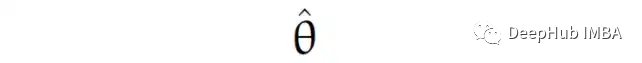0

# MSE = Bias² + Variance?什么是“好的”统计估计器

“偏差-方差权衡”是ML/AI中被经常提到的一个流行概念。我们这里用一个直观的公式来对它进行解释:

MSE = Bias² + Variance## E(X)和V(X)E (X) = (1) + (1/6) (2) (1/6) + (3) (1/6) + (4) (1/6) + (5) (1/6) + (6) (1/6) = 3.5

`````` V(X) = E[(X - E(X))²] = ∑[x - E(X)]² P(X = x)
``````

`````` V(X) = ∑[x - E(X)]² P(X=x) = ∑(x - 3.5)² P(X=x) = (1–3.5)² (1/6) + (2–3.5)² (1/6) + (3–3.5)² (1/6) + (4–3.5)² (1/6) + (5–3.5)² (1/6) + (6–3.5)² (1/6) = 2.916666…
``````

V(X)公式的另外一个备选

`````` V(X) = E[(X - E(X))²] = E[(X )²] - [E(X)]²
``````

`````` V(X) = E[(X - E(X))²]
= E[X² - 2 X E(X) + E(X)²]
= E(X²) - 2 E(X) E(X) + [E(X)]²
= E[(X )²] - [E(X)]²
``````

## 关于符号的注释

Estimand（你想要估计的东西，估计目标或者叫被估计值）通常用朴素的希腊字母表示，最常见的是 θ。（“th”应该有自己的字母，这就是我们在英语中使用的字母“theta”；“th”足够接近“pffft”，使 θ 成为统计学中标准占位符的真正绝佳选择 .)## 推导MSE公式

Estimand：θ，我们要估计的东西，一个常数。

Estimator，我们用来获得估计值的公式，它是一个取决于你获得的数据的随机变量。

Estimate ：θ_hat，一旦我们将数据送入估计器，最后就会出现一些数字，这就是估计。

`````` E(X) = E((θhat - θ)) = E(θhat ) - E(θ) = E(θhat) - E(θ) = E(θhat) - θ
``````

Bias = E(θhat) - θ

## 选择“最佳”估计器

`````` 方差 V(X) = E[(X)²] - [E(X)]²
变为 V(θhat -θ) = E[(θhat - θ)²] - [E(θhat - θ)]²
``````

``````     V(θhat) = E[(θhat - θ)²] - [E(θhat) - E(θ)]²
``````

``````     E[(θhat - θ)²] = [E(θhat) - θ]² + V(θhat)
``````

``````     Bias = E(θhat) — θ
``````

``````     E[(θhat - θ)²] = [Bias]² + V(θhat) = Bias² + Variance
``````

E(ε²)又被称作为均方误差！简称 MSE。它的字面意思是 E(ε²)：我们取均方误差 ε² 的平均值（期望值的另一个词）。MSE 是模型损失函数最流行的（也是普通的）选择，而且它往往是我们学习的第一个损失，所以我们就得到了：

MSE = Bias² + Variance

## 总结

### “MSE = Bias² + Variance?什么是“好的”统计估计器”的评论:

##### 关于作者##### Deephub